### Stress: First and Second Piola-Kirchhoff Stress Tensors

### Definitions

The Cauchy stress tensor defined previously, related area vectorsto traction vectors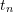in the current state of deformation of a material object. The first and second Piola-Kirchhoff stress tensors extend the concept of “true” and “engineering” stress to the three-dimensional case and operate on area vectors in the undeformed state of the material. Assume that the area vector before any forces are applied to a continuum is equal to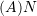where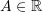is the magnitude of the area and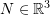is the vector perpendicular to the underformed area. Assume that the forces applied lead to a deformation described by the linear mapping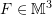such that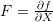where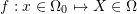. Let the area vector after deformation be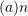where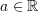is the magnitude of the deformed area and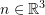is the vector perpendicular to the deformed area. Then, using Nanson’s formula: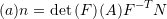The blue vectors in Figure 1 show a schematic of two undeformed unit area veoctors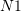and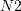(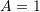) and their respective images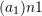and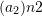after transformation by a linear mapping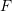. After deformation, the force vector acting on an area with normaland magnitudeis equal to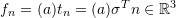. The first Piola-Kirchhoff stress tensor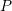is defined as the tensor producing the same force vector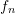when applied to the corresponding undeformed area vector: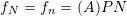Using Nanson’s formula: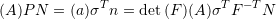Therefore,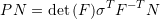Which results in the following relationship betweenand: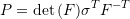Note that the above relationship indicates thatis not necessarily a symmetric matrix. The term “Nominal Stress Tensor” is sometimes used in the literature in reference to the first Piola-Kirchhoff stress or its transpose. The red vectors in Figure 1 show a schematic of the forces acting on the deformed area vectorsandwhen viewed in the deformed configuration or when viewed in the undeformed configuration.
On the other hand, the second Piola-Kirchhoff stress tensor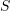is defined as the tensor producing a force vector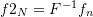when applied to the undeformed area vector.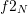is sometimes termed the “pull-back” of the force vector. The relationship betweenand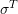can be obtained as follows: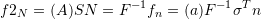Therefore: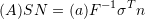Using Nanson’s formula: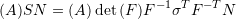Which results in the following relationship betweenand: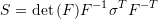Thereforeis a symmetric tensor!
Notice that the relationship betweenandis: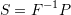The black vectors in Figure 1 show a schematic of the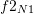and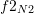which are the “pull-back” of the force vectors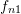and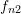.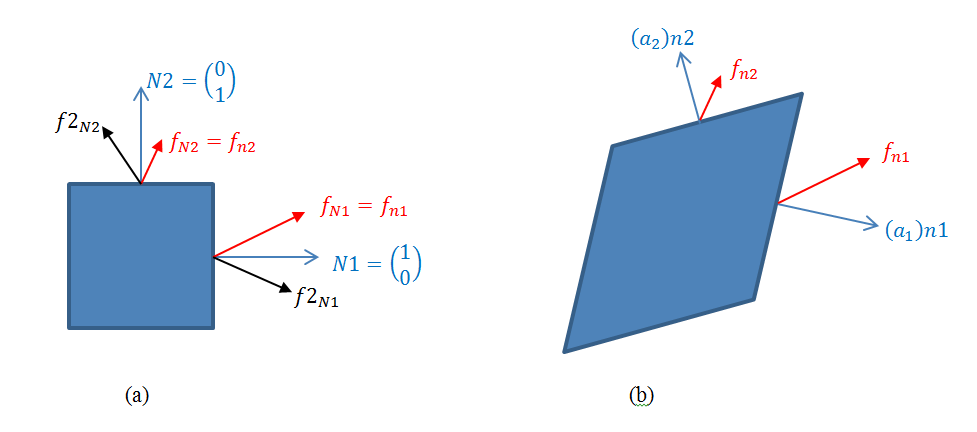Figure 1. The force and area vectors on (a) the undeformed configuration, and (b) the deformed configuration.

The illustrative examples 1, 2, and 3 in the energy section can help clarify the difference between the three major stress tensors.

#### Example

Consider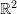. Assume that a region inside a material deforms according to the linear transformation described by: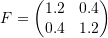Assume that the stress in the deformed configuration is described by the matrix: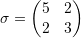Find:

• The area vectors after deformation of the two original unit areas with area vectors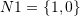and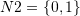.
• The first and second Piola-Kirchhoff stress matrices.
• The force vectors on the deformed planes whose undeformed unit area vectors areandusing the Cauchy stress matrix.
• The force vectors produced by applying the first Piola-Kirchhoff stress tensor on the undeformed unit area vectorsand.
• The force vectors produced by applying the second Piola-Kirchhoff stress tensor on the undeformed unit area vectorsand.
##### Solution

Using Nanson’s formula (with):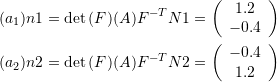Where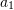and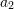are the magnitudes of the “deformed” areas and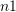and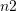are the corresponding normal vectors to the areas whose original normal vectors before deformation areand, respectively. The first and second Piola-Kirchhoff stress matricesandare: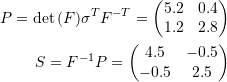The force vectors on the deformed area vectorsandare: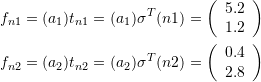The force vectors on the original (undeformed) area vectorsandobtained using the first Piola-Kirchoff stress are exactly the same ():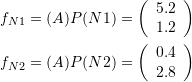The force vectors on the original area vectors N1 and N2 when using the Second Piola-Kirchhoff stress ():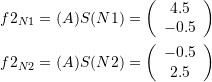Notice that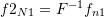and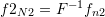. Figure 1 shows the deformation of an originally square object of unit length and the corresponding deformation of areas. The force vectors are also shown on the different areas before and after deformation. The Cauchy stress and the first Piola-Kirchhoff stress tensors produce the same
force vectors. The Cauchy stress matrix can intuitively be viewed as the force in the deformed configuration per unit area of the deformed configuration. The first Piola-Kirchhoff stress matrix can intuitively be viewed as the force in the deformed configuration per unit area of the undeformed configuration, while the second Piola-Kirchhoff stress can intuitively be viewed as the force in the undeformed configuration per unit area of the undeformed configuration. Notice that all these stress measures are equivalent, but they are useful for different applications.

View Mathematica Code:
F={{1.2,0.4},{0.4,1.2}};
Bx=Rectangle[{0,0}];
Bx2=GeometricTransformation[Bx,F];
Sigma={{5,2},{2,3}};
N1={1,0};
N2={0,1};
Farea=Det[F]*Transpose[Inverse[F]];
an1=Farea.N1;
an2=Farea.N2;
tn1=Transpose[Sigma].an1
tn2=Transpose[Sigma].an2
FirstPiola=Transpose[Sigma].Inverse[Transpose[F]]*Det[F];
SecondPiola=Inverse[F].FirstPiola;
TN1=FirstPiola.N1;
TN2=FirstPiola.N2;
STN1=SecondPiola.N1;
STN2=SecondPiola.N2;
Pt11=F.N1+1/2*F.N2;
Pt12=Pt11+an1;
Pt21=1/2*F.N1+F.N2;
Pt22=Pt21+an2;
ar1=Arrow[{Pt11,Pt12}];
ar2=Arrow[{Pt21,Pt22}];
A1dotted=Arrow[{Pt11,Pt11+{1,0}}];
A2dotted=Arrow[{Pt21,Pt21+{0,1}}];
A1=Arrow[{{1,0.5},{2,0.5}}];
A2=Arrow[{{0.5,1},{0.5,2}}];
artn1=Arrow[{Pt11,Pt11+1/10*tn1}];
artn2=Arrow[{Pt21,Pt21+1/10*tn2}];
arTN1=Arrow[{{1,0.5},{1,0.5}+1/10*TN1}];
arTN2=Arrow[{{0.5,1},{0.5,1}+1/10*TN2}];
arSTN1=Arrow[{{1,0.5},{1,0.5}+1/10*STN1}];
arSTN2=Arrow[{{0.5,1},{0.5,1}+1/10*STN2}];
Graphics[{Black,Bx,A1,A2,GrayLevel[0.1],arTN1,arTN2,GrayLevel[0.5],arSTN1,arSTN2}]
Graphics[{Black,Bx2,ar1,ar2,GrayLevel[0.1],artn1,artn2,Dashed,GrayLevel[0.5],A1dotted,A2dotted}]


In the following tool, change the values ofandand watch how they affect the resulting deformation and forces acting on the different faces.